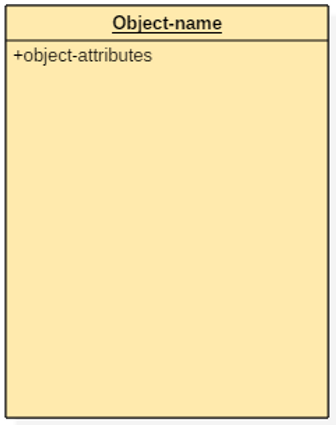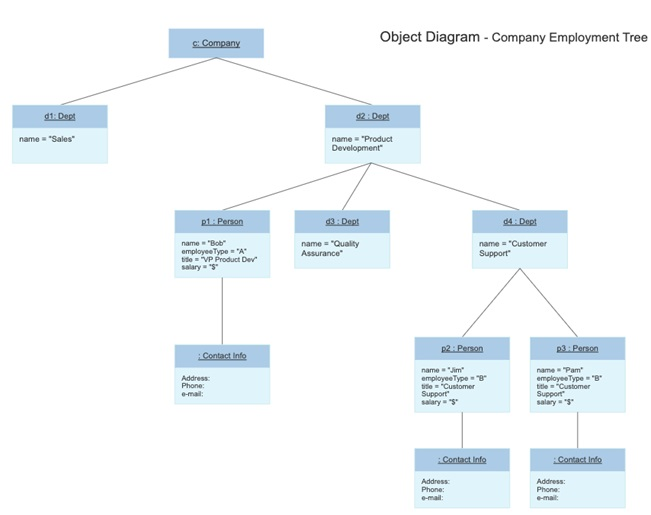# Object Diagram in Unified Modeling Language (UML)

In this tutorial, we will learn about the object diagram in unified modeling language (UML), its notations, and examples. By Monika Sharma Last updated : April 05, 2023

## What is an Object Diagram in UML?

An object is a real-world problem entity whose activities are defined by the classes. The Object is a specimen of a particular moment in runtime, plus objects and data ethics or values. A steady UML object diagram is an example of a class diagram; it shows a snap of the detailed state of a system at a point in time, thus an object diagram circumscribe objects and their relationships at a point in time. It may examine a special case of a class diagram or a communication diagram.

## Notations of Object Diagram

The notations of object diagrams are similar to the class diagrams.

### 1) Name

Every object is represented like a rectangle, that offers the name from the object and its class emphasize as well as divided with a colon.

### 2) Attributes

Similar to classes, you can list object characteristics inside a separate section. However, unlike classes, object credit should have values given to them.

Links tells to be an example associated with the relationship. You can draw a link while using the lines utilized in class diagrams.## Motive of Object Diagram

The object diagrams are used for the following purposes:

1. It is used to represent the occurrence of the class. You might create a class diagram to present the structure and then create a set of objectives.
2. It is used to understand the activity or working of an object.

In summary, the relation between the instantiated class and the defined class is been shown by the Object diagram. The best way to exemplify what an object diagram looks like is to show the object diagram generated from a similar class diagram.

## Steps for Drawing an Object Diagram

A communication diagram called collaboration in the previous version of UML without comment is known as an object diagram. An object diagram must be a valid actualization of a fixed class diagram. This can be used as a fast regularity check. To do this we can develop an object diagram by using the following steps:

• Recognize the mechanism you'd like to draw. An apparatus constitute some functions or behaviors of the part of the system you are modeling.
• Show the state and accredit values of each such object, as necessary, to understand the scene.

## Instance Specification of an Object Diagram

Each object and link on an object diagram is presented by a current quality. This can show an object's classifier e.g. an abstract or concrete class and instance name, as well as attributes and other structural features using slots. Each part corresponds to a single meaning or feature and may include a value for that state.

The name on an instance specification optionally shows an instance name, separator, and not compulsory one or more classifier names separated by commas.

## Object Diagram ExampleImage source: https://cloud.smartdraw.com/editor.aspx?templateId=680993af-b8b1-48a9-8fce-3f57a65eb834&noro=1&nsu=1